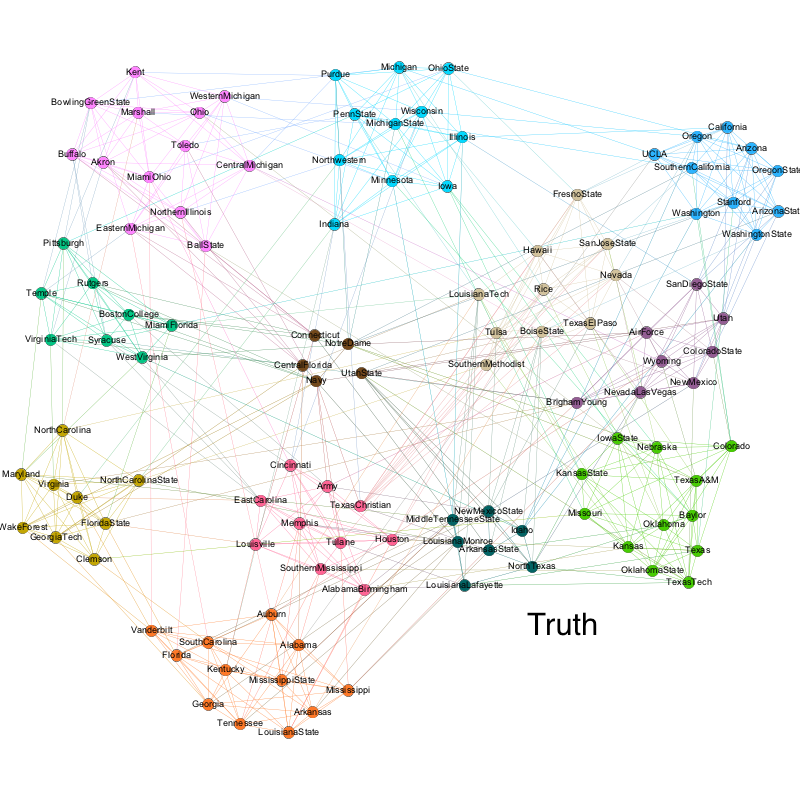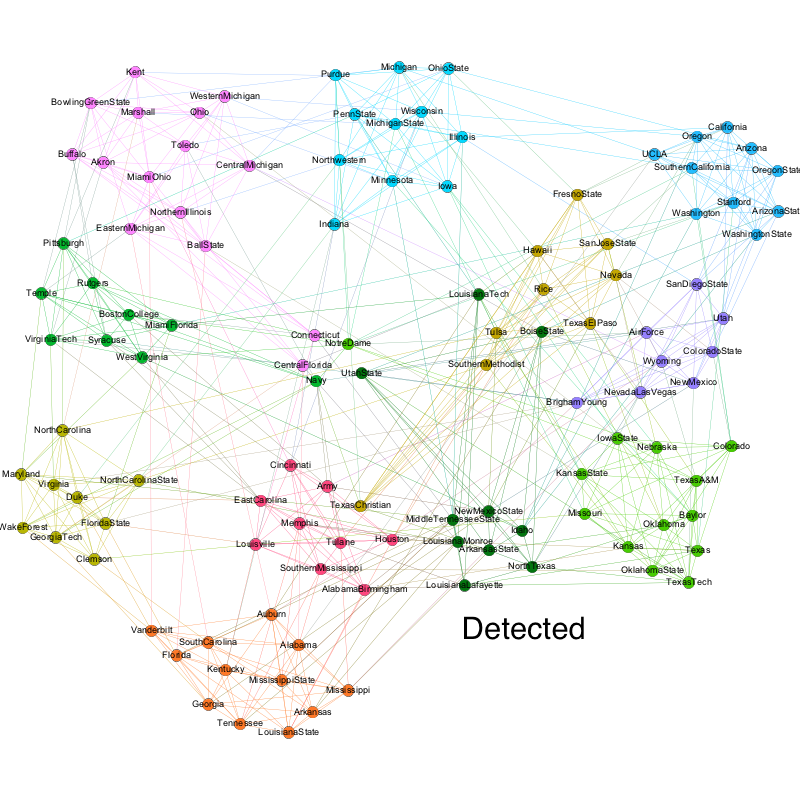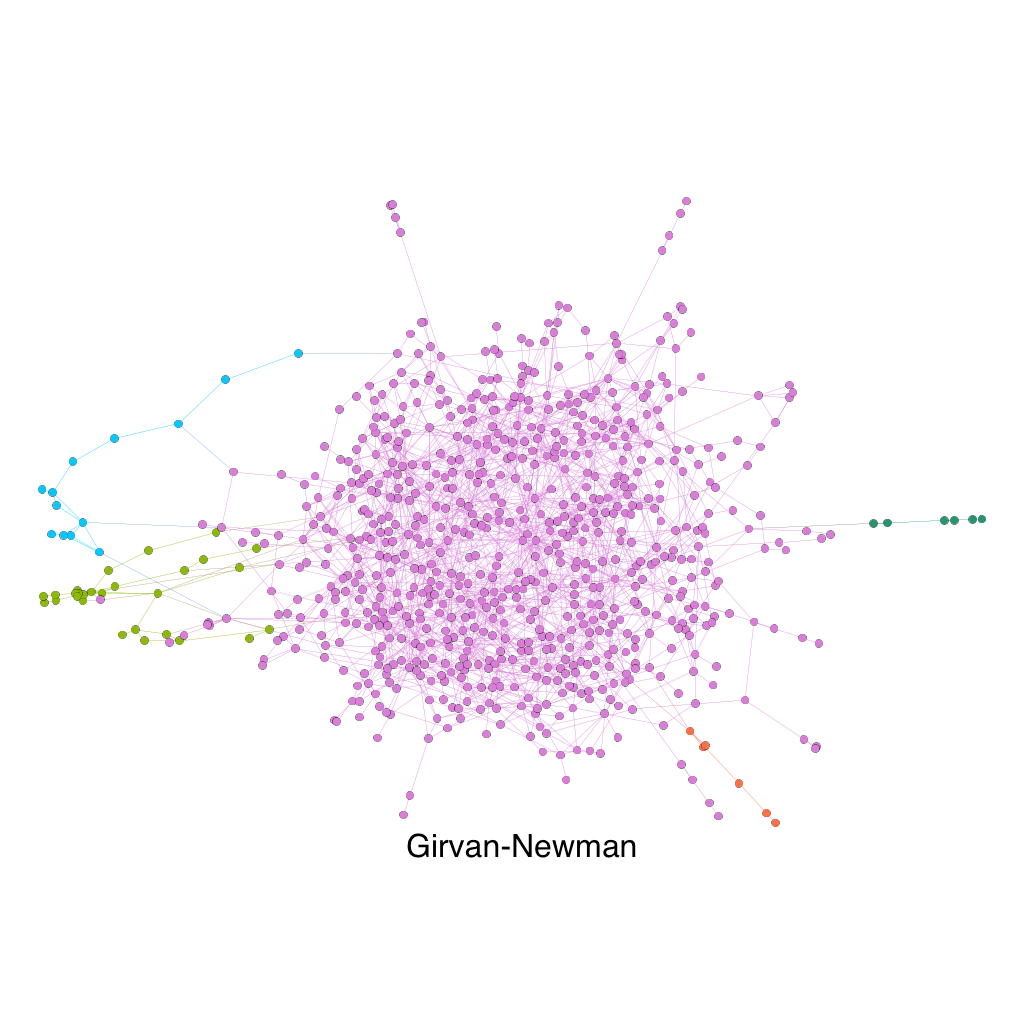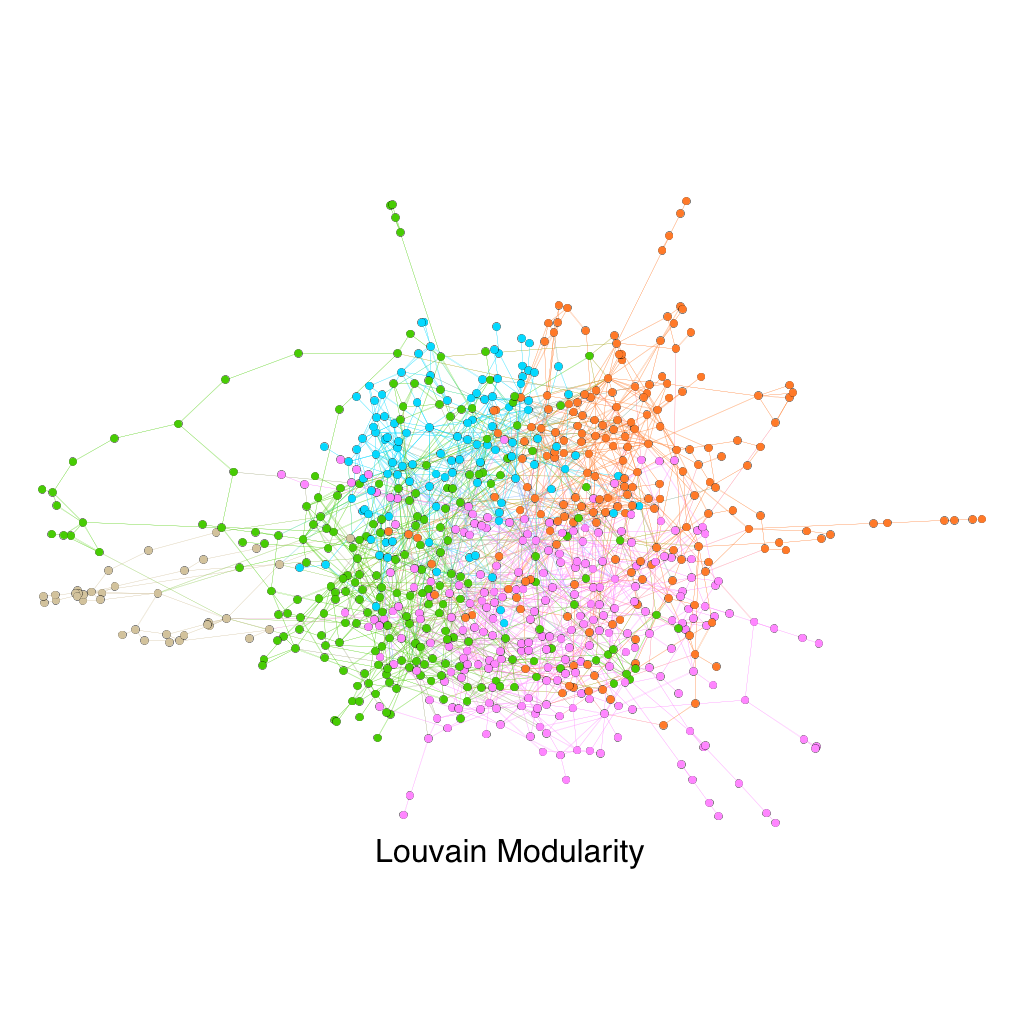# Detecting Community Structures in Networks

The purpose of this post is two-folded

## Problem

A well known property in networked systems is that network nodes are joined together in tightly knit groups. The connections within groups are significantly denser compared with connections between different groups. The Girvan-Newman algorithm is an method for detecting such close knit communities, leveraging the idea of betweenness of edges. My goal here is to implement the Girvan-Newman algorithm in java, and apply it to a few datasets to observe how it performs.

## Data

When it comes to implementing an algorithm from a paper, you can’t get better datasets other than the ones used by the original authors. Therefore I picked two datasets that was from the original paper. I also applied the algorithm on a slightly larger graph(~1000 nodes) just to see how it performs on larger graphs that has no clear communities.

• Zachary’s Karate Club: A 34 node graph of Social network of friendships between 34 members of a karate club at a US university in the 1970
• American College Football: A 114 node graph of network of American football games between Division IA colleges during regular season Fall 2000
• UCSD Facebook Graph - 1000: A scaled-down version (783 nodes) of the Facebook friendships between students at UCSD in 2005

## Algorithm

The key concepts in the Girvan-Newman Algorithm is edge betweenness, which is defined as the number of shortest paths between pairs of vertices that run along it. If there’s more than one shortest path between a pair of vertices, each path is given equal weight. The interpretation behind the edge betweenness is that communities in the network are loosely connected by a few edges, hence all the shortest paths between the communities would have go through one of these few edge, which results in high edge betweenness. The next step is to remove the edge with the highest betweenness and re-compute the betweenness for all the edges. We iterate this until a desired number of communities has already been reached or until there is no edge remain.

The first problem I encountered was to find all the shortest paths between a pair of vertices. We could easily find one shortest path with Bread First Search (BFS), but it would return the path immediately after it finds one, instead of finding all shortest paths. Hence, I needed to make some minor modifications to the BFS algorithm. The two key modification is as follows

• Allow vertices to be discovered more than once from different vertices, as long as the vertex getting discovered is one level further than the vertex from which it discovers
• Allow vertices to have multiple parents
• After finding the target node, run Depth First Search (DFS) through the parents all the way to the starting vertex to return all possible shortest paths

### Finding Number of Communities In the Network

There is a well-known algorithm for finding all Strongly Connected Components in a graph. The number of Strongly Connected Components(SCC) is the number of communities in the graph. The algorithm is Tarjan’s strongly connected components algorithm

### Sidenote

It was pointed out from the original paper that when recalculating the betweenness of all edges, only the betweenness of the edges that are affected by the removal of the edge with highest betweenness would need to get re-computed. This may improve the time complexity of the algorithm, which is the main disadvantage of the algorithm. The time complexity is (O(m2n)), where m is the number of edges and n is the number vertices. However, this could dramatically increase the space complexity. Since there is no way of knowing which edge would be removed before hand, we would need to store the shortest path between all pairs of vertices. Best case scenario, all pairs of vertices has constant shortest path, which gives (O(n2)), which is already greater than the space we needed for the adjacency list we need to store the graph. The worst case of number of shortest path between a pair of vertices would be exponential!. So I don’t think this is a good idea, as the worst case would most certainly kill the algorithm.

## Results

### Zachary’s Karate Club

The graph below shows the two community separation made by the Girvan-Newman algorithm, compared with the ground-truth communities. The only misclassified node is marked green, which is node 3 in the above graph. As we can see, the algorithm performed pretty well and the result is consistent with the result from the original paper.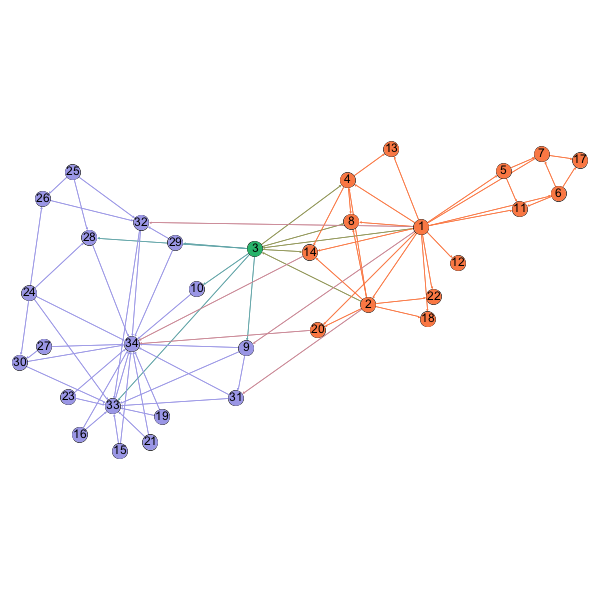### American College Football

The two graphs below shows the comparison between the communities detected based on the American football games between Division IA colleges and the ground truth conferences each college plays in. As we can see, the algorithm did a great job, which is consistent with the results from the original paper. It is worth noting that since the algorithm had a difficult detecting the “Independent” schools, which are the brown cluster in the middle. This is because independent schools aren’t really a league and they play against schools in other conferences, as opposed to other independent schools.